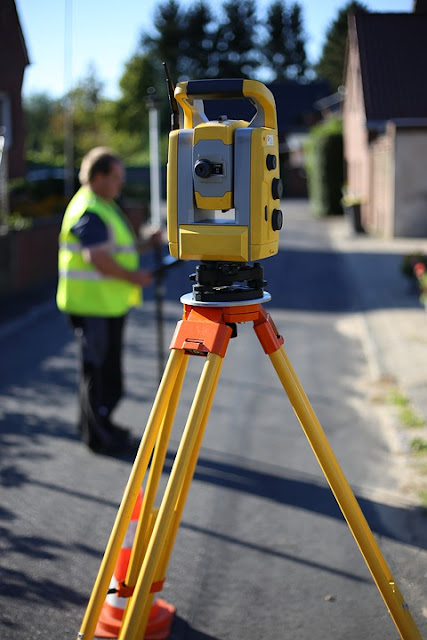# Basic Concepts of Surveying | Part 2 | Introduction to Surveying | Explanatory Video | Surveying and Geomatics | By Ashutosh Nautiyal

Principles of Surveying We do the Reconnaissance (Recy Survey) to have an idea of the area to be surveyed. We establish the Control Network so that it controls the survey. We work from whole to part to avoid the accumulation of the errors. While we are plotting the objects on the map, we need to choose the scale and the scale of an area is decided by the size of that area and the significance of the work.

## Principles of Surveying

• We do the Reconnaissance (Recy Survey) to have an idea of the area to be surveyed.

• We establish the Control Network so that it controls the survey.

• We work from whole to part to avoid the accumulation of the errors.

• While we are plotting the objects on the map, we need to choose the scale and the scale of an area is decided by the size of that area and the significance of the work.

• We should have redundancy in the measurement or we should go for the check line to check the accuracy of the Surveying.

• If the size of objects, features or separation between two objects is less than the plotting accuracy in the ground, then we can't show it on the map.
Plotting accuracy = 0.25 mm * n
where n = 1/ScaleBasic Concepts of Surveying (Part 2)

## Scale

The scale is represented in three ways:-

## Representative Fraction (RF)

• RF is represented as:-

• RF = 1:n

• RF (1:n) signifies that 1 unit on the map is equal to n units on the ground.

• RF = 1:1000 means that 1 cm on the map is equal to 1000 cm on the ground.

• If RF is large, the scale is large and if RF is small, the scale is small.

## Engineer's Scale

• Engineer's Scale is represented as:-

• 1 cm = 50 m

• It signifies that 50 m on the ground is equivalent to 1 cm on the map.

## Graphical Scale

• Graphical Scale is nothing but a graphical way of showing the scale.

• The graphical scale is plotted on the map in the form of a line.

• There is one advantage with the graphical scale which is like this:-

• If the map shrinks or expands with a long period of time (like 10 years or more than that) then the graphical scale will also have the same amount of shrinkage or expansion. So, because of this, we won't be facing any error in the measurement while using the graphical scale.

## Classification of Survey

It is done on the following bases:-

• On the basis of the type of Surveying (Plane or Geodetic Surveying)

• On the basis of Area (like a town, a village, an agricultural area, etc.)

• On the basis of Equipment (like a chain, tape, compass, theodolite,  GPS, etc.)

• On the basis of Technique (like triangulation, traverse method, etc.)

• On the basis of Function (like a military survey, an agricultural survey, hydrographic survey, etc.)

*Self Typed
*Source - Internet, Books, Self-Analysis

Getting Info...